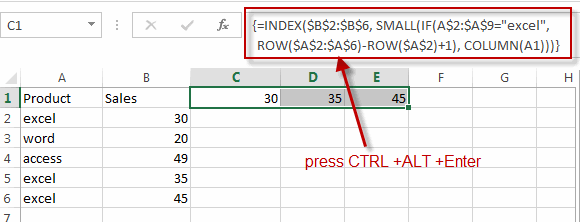# VLOOKUP Return Multiple Values Horizontally

This post will guide you how to vlookup a value and then return multiple corresponding values horizontally in Excel. How do I lookup a value and return multiple matched values in the same row in Excel. Or how to return multiple values with VLOOKUP function in Excel.

## VLOOKUP Return Multiple Values Horizontally

Assuming that you have a list of data that contain product name and product sales in the range A1:B6, and you want to vlookup a product “excel”” and return all sales value of the “excel” horizontally.

We have talked that the VLOOKUP function can be used to return only a corresponding value of the first occurrence of a lookup value. And if you want to return multiple values horizontally, how to achieve it.

You can create a complex array formula based on the INDEX function, the SMALL function, the IF function, the ROW function and the COLUMN function.

`=INDEX(\$B\$2:\$B\$6, SMALL(IF(A\$2:\$A\$9="excel", ROW(\$A\$2:\$A\$6)-ROW(\$A\$2)+1), COLUMN(A1)))`

Type this formula into a blank cell and then press Ctrl+Alt+Enter shortcuts to change this formula as array formula. And then drag the AutoFill handle from Cell C1 to E1.You will see that the multiple values have been extracted into the same row.

### Related Functions

• Excel SMALL function
The Excel SMALL function returns the smallest numeric value from the numbers that you provided. Or returns the smallest value in the array.The syntax of the SMALL function is as below:=SMALL(array,nth) …
• Excel INDEX function
The Excel INDEX function returns a value from a table based on the index (row number and column number)The INDEX function is a build-in function in Microsoft Excel and it is categorized as a Lookup and Reference Function.The syntax of the INDEX function is as below:= INDEX (array, row_num,[column_num])…
• Excel ROW function
The Excel ROW function returns the row number of a cell reference.The ROW function is a build-in function in Microsoft Excel and it is categorized as a Lookup and Reference Function.The syntax of the ROW function is as below:= ROW ([reference])….
• Excel IF function
The Excel IF function perform a logical test to return one value if the condition is TRUE and return another value if the condition is FALSE. The IF function is a build-in function in Microsoft Excel and it is categorized as a Logical Function.The syntax of the IF function is as below:= IF (condition, [true_value], [false_value])….
• Excel COLUMN function
The Excel COLUMN function returns the first column number of the given cell reference.The syntax of the COLUMN function is as below:=COLUMN ([reference])….
• Excel VLOOKUP function
The Excel VLOOKUP function lookup a value in the first column of the table and return the value in the same row based on index_num position.The syntax of the VLOOKUP function is as below:= VLOOKUP (lookup_value, table_array, column_index_num,[range_lookup])….